# NCERT Solutions for Class 6 Maths Chapter 3 Playing With Numbers Ex 3.7

These NCERT Solutions for Class 6 Maths Chapter 3 Playing With Numbers Ex 3.7 Questions and Answers are prepared by our highly skilled subject experts.

## NCERT Solutions for Class 6 Maths Chapter 3 Playing With Numbers Exercise 3.7

Question 1.
Renu purchases two bags of fertilizer of weights 75 kg and 69 kg. Find the maximum value of weight which can measure the weight of the fertilizer exact number of times.
For finding maximum weight, we have to find H.C.F. of 75 and 69.
Factors of 75 = 3 × 5 × 5
Factors of 69 = 3 × 23
H.C.F. = 3
Therefore, 3 kg is the maximum value of weight which can measure the weight of the fertiliser exact number of times.Question 2.
Three boys step off together from the same spot. Their steps measure 63 cm, 70 cm and 77 cm respectively. What is the minimum distance each should cover so that all can cover the distance in complete steps?
For finding minimum distance, we have to find L.C.M of 63, 70 and 77.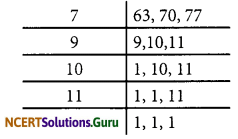L.C.M. of 63, 70 and 77
= 7 × 9 × 10 × 11 = 6930 cm.
Therefore, the minimum distance is 6930 cm.

Question 3.
The length, breadth and height of a room are 825 cm, 675 cm and 450 cm respectively. Find the longest tape which can measure the three dimensions of the room exactly.
The measurement of longest tape = H.C.F. of 825 cm, 675 cm and 450 cm. Factors of 825 = 3 × 5 × 5 × 11 Factors of 675 = 3 × 5 × 5 × 3 × 3
Factors of 450 = 2 × 3 × 3 × 5 × 5
H.C.F. = 3 × 5 × 5 = 75 cm
Therefore, the longest tape is 75 cm.

Question 4.
Determine the smallest 3-digit number which is exactly divisible by 6, 8 and 12.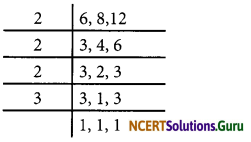L.C.M. of 6, 8 and 12
= 2 × 2 × 2 × 3 = 24
The smallest 3-digit number =100
To find the number, we have to divide 100 by 24
100 = 24 × 4 + 4
Therefore, the required number = 100 + (24 – 4) = 120.Question 5.
Determine the greatest 3-digit number which is exactly divisible by 8,10 and 12.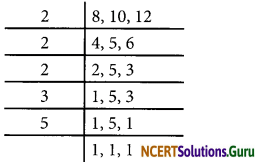L.C.M. of 8,10, 12 = 2 × 2 × 2 × 3 × 5 = 120
The largest three digit number = 999
To find the number, we have to divide 999 by 120
999 = 120 × 8 + 39
Therefore, the required number = 999 – 39 = 960

Question 6.
The traffic lights at three different road crossings change after every 48 seconds, 72 seconds and 108 seconds respectively. If they change simultaneously at 7 a.m. at what time will they change simultaneously again?L.C.M. of 48, 72, 108
= 2 × 2 × 2 × 2 × 3 × 3 × 3 = 432 seconds
After 432 seconds, the lights change simultaneously.
432 seconds = 7 minutes 12 seconds
Therefore, the time = 7 a.m. + 7 minutes 12 seconds = 7:07:12 a.m.

Question 7.
Three tankers contain 403 litres, 434 litres and 465 litres of diesel respectively. Find the maximum capacity of a container that can measure the diesel of three containers exact number of times.
The maximum capacity of container = H.C.F. (403, 434, 465)
Factors of 403 = 13 × 31
Factors of 434 = 2 × 7 × 31
Factors of 465 = 3 × 5 × 31
H.C.F. = 31
Therefore, 31 litres of container is required to measure the quantityQuestion 8.
Find the least number which when divided by 6, 15 and 18, leave remainder 5 in each case.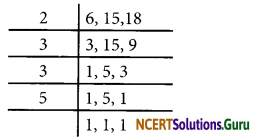L.C.M. of 6, 15 and 18
= 2 × 3 × 3 × 5 = 90
Therefore, the required number.
= 90 + 5 = 95.

Question 9.
Find the smallest 4-digit number which is divisible by 18, 24 and 32.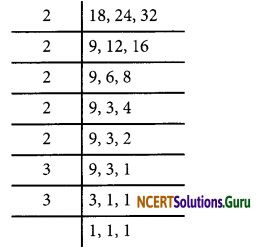L.C.M. of 18, 24 and 32
= 2 × 2 × 2 × 2 × 2 × 3 × 3 = 288
The smallest four-digit number = 1000
To find the number, we have to divide 1000 by 288
1000 = 288 × 3 + 136
Therefore, the required number is 1000+ (288 – 136) = 1152.

Question 10.
Find the L.C.M. of the following numbers:
(a) 9 and 4
(b) 12 and 5
(c) 6 and 5
(d) 15 and 4
Observe a common property in the obtained L.C.Ms. Is L.C.M. the product of two numbers in each case?
(a) L.C.M. of 9 and 4
= 2 × 2 × 3 × 3 = 36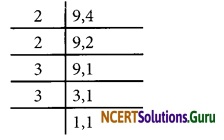(b) L.C.M. of 12 and 5 = 2 × 2 × 3 × 5 = 60(c) L.C.M. of 6 and 5 = 2 × 3 × 5 = 30 6,5(d) L.C.M. of 15 and 4 = 2 × 2 × 3 × 5 = 60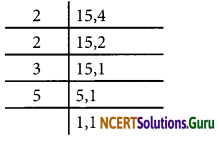Yes, L.C.M. is equal to the product of rbers in each case. And L.C.M. is multiple of 3.

Question 11.
Find the L.C.M. of the following numbers in which one number is the factor of other:
(a) 5,20
(b) 6,18
(c) 12,48
(d) 9,45
What do you observe in the result obtained?
(a) L.C.M. of 5 and 20 = 2 × 2 × 5 = 20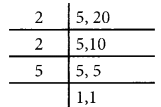(b) L.C.M. of 6 and 18 = 2 × 3 × 3 = 18(c) L.C.M. of 12 and 48 = 2 × 2 × 2 × 2 × 3 = 48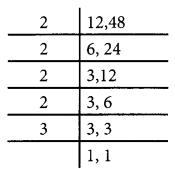(d) L.C.M. of 9 and 45 = 3 × 3 × 5 = 45From these all cases, we can conclude that if the smallest number if the factor of largest number, then the L.C.M. of these two numbers is equal to that of larger number.

error: Content is protected !!